出租广告位,需要合作请联系站长

+关注

debug(0)

2021-08(3)

2021-09(11)

# 主要内容:

## 认识异常

### 异常的基本概念

``````a = 10
prin(a)  # NameError: name 'prin' is not defined
``````

``````a = [1,2,3]
print(a)	#IndexError: list index out of range
``````

``````a = open('Z:/zzz.txt','r')
#FileNotFoundError: [Errno 2] No such file or directory: 'Z:/zzz.txt'
``````

#### 总结：

• 异常是指程序执行过程中, 发生了错误. 根据不同的错误类型, 抛出不同类型的异常.
• 如果一个程序抛出了异常, 如果不对这个异常做任何处理, 默认行为就是导致程序运行终止.

C语言如何处理”异常“

### 处理异常

##### try捕捉异常

except可以指定一个具体的处理哪种类型异常. 如果try中抛出的异常类型, 不在except中, 那么仍然会程序终止.

IndexError, e相当于捕捉到的这个异常对象的名字为e. 这个异常对象中包含了异常的具体信息.这个e相当于这个异常类的实例

``````a = [1,2,3]
try:
print(a)
print('hello')
except IndexError as e:
print(type(e))
print(e)
#执行结果：
<class 'IndexError'>
list index out of range

#如果把hello放上面:
a = [1,2,3]
try:
print('hello')
print(a)
except IndexError as e:
print(type(e))
print(e)
#执行结果：
hello
<class 'IndexError'>
list index out of range
``````

##### try和except配合

``````a = [1,2,3]
try:
print(a)
except IOError as e:
print(e)
except IndexError as e:
print(e)
#执行结果
list index out of range
``````

##### try和else配合

``````a = [1,2,3]
try:
print(a)
except IOError as e:
print(e)
except IndexError as e:
print(e)
else:
print("程序没有异常")
#执行结果
1

``````

except可以不带具体的异常类型, 这样只要try中触发了任意的异常, except都能捕捉到

``````a = [1,2,3]
try:
print('hello')
print(a)

except :
print("捕获到异常")
#执行结果：
hello

``````

##### 异常的基类:Exception类

Python中内置的异常类, 其实都继承自 Exception 类. 所以上面的代码还可以写成

``````a = [1,2,3]
try:
print(a)
except Exception as e:
print(e)
else:
print("程序没有异常")
#执行结果：
list index out of range
``````

##### finally

``````a = [1,2,3]
try:
print(a)
except Exception as e:
print(e)
finally:
print('End')
#执行结果
list index out of range
End
``````

with语句的本质就是try…finally

``````try:
f = open('Z:/test.txt','r')
for line in f:
print(line.strip())
except Exception as e:
print('异常:',' ')
print(e)
finally:
print('End')
f.close()	#无论try是否触发异常,都会调用finally中的f.close()
#执行结果
Mango
hello
world
End
``````

### 抛出异常

##### raise关键字

``````def Divide(x,y):
if y == 0:
raise Exception('divide zero')  #除数为0	用异常类创建一个异常对象
return x/y
Divide(1,0)
#执行结果：
Exception: divide zero
``````

``````def Divide(x,y):
if y == 0:
raise Exception('divide zero')  #除数为0
return x/y
#手动捕获异常
try:
Divide(1,0)
except Exception as e:
print(e)
#执行结果：
divide zero
``````

### 内置异常类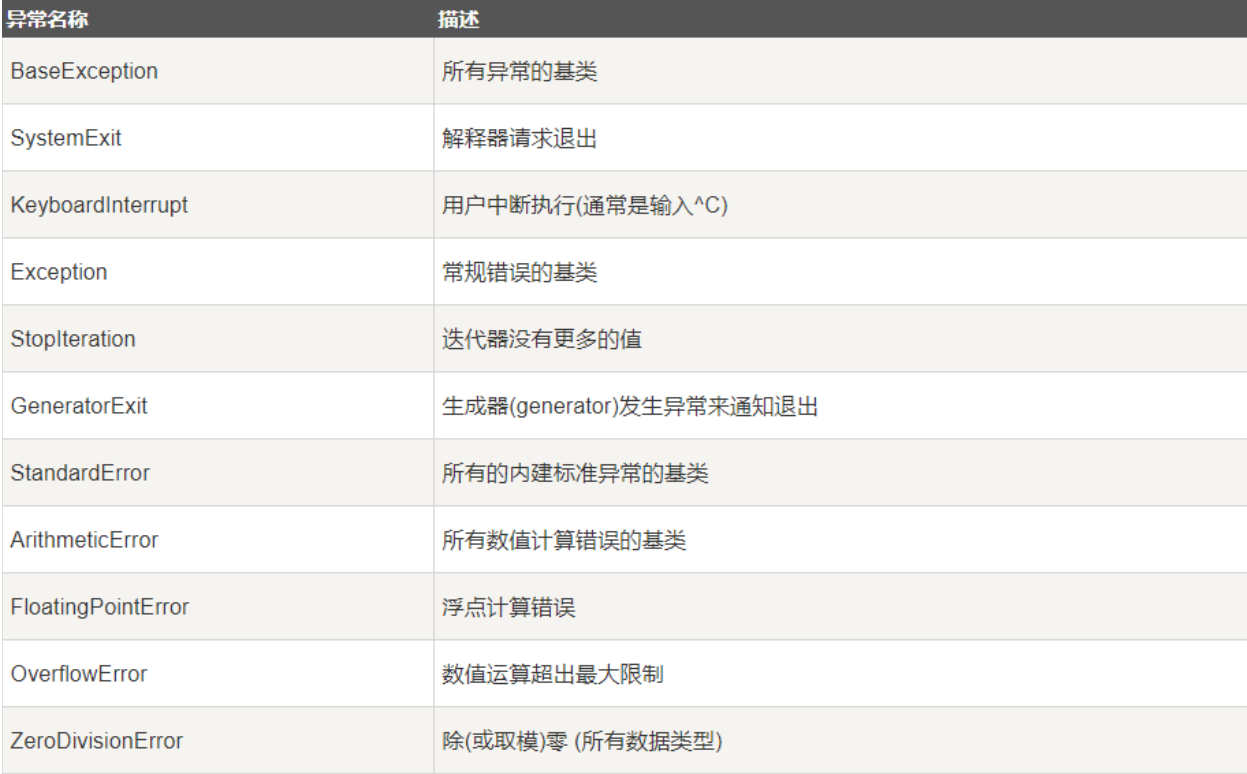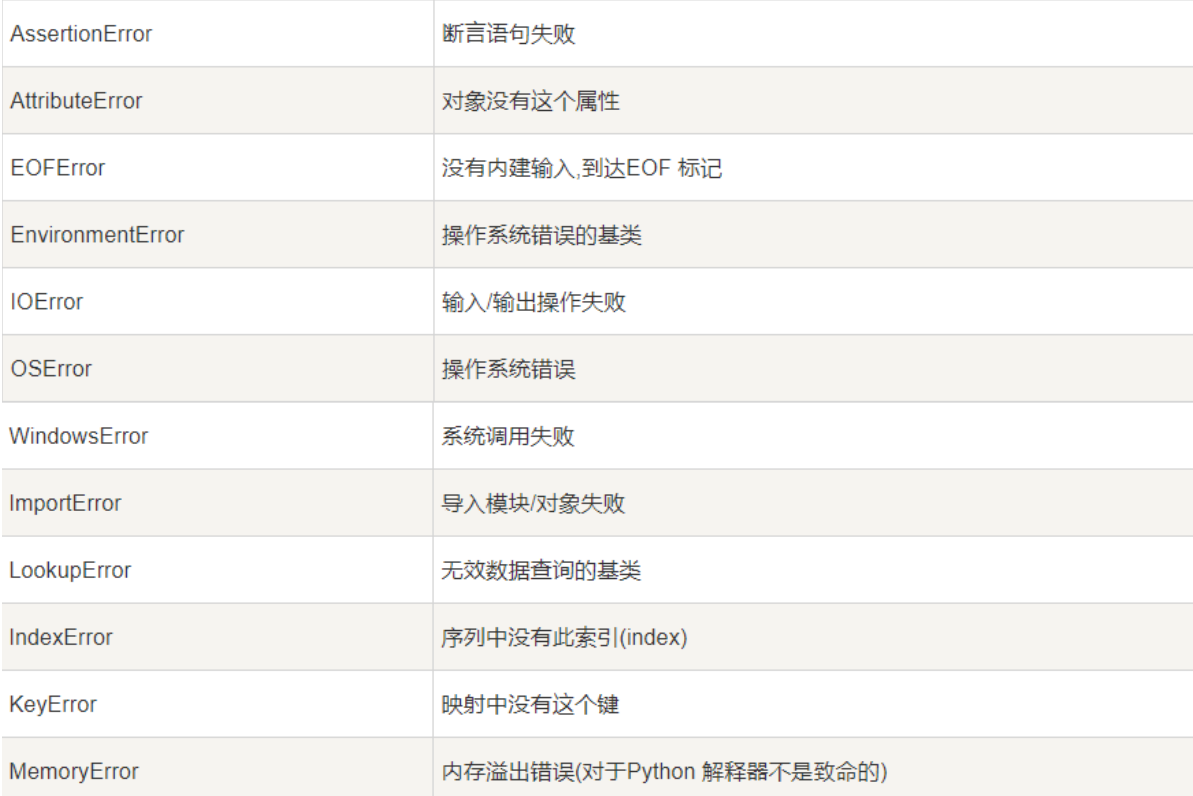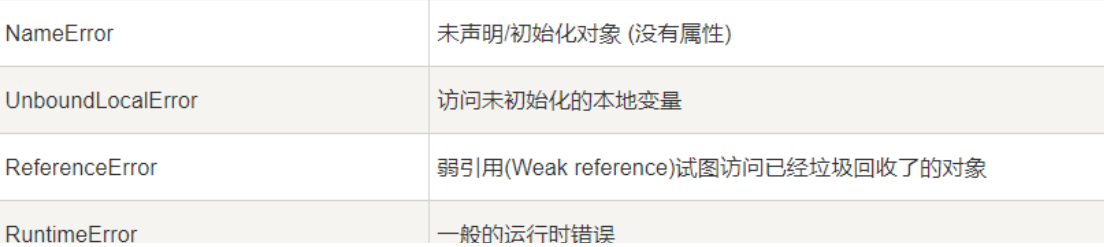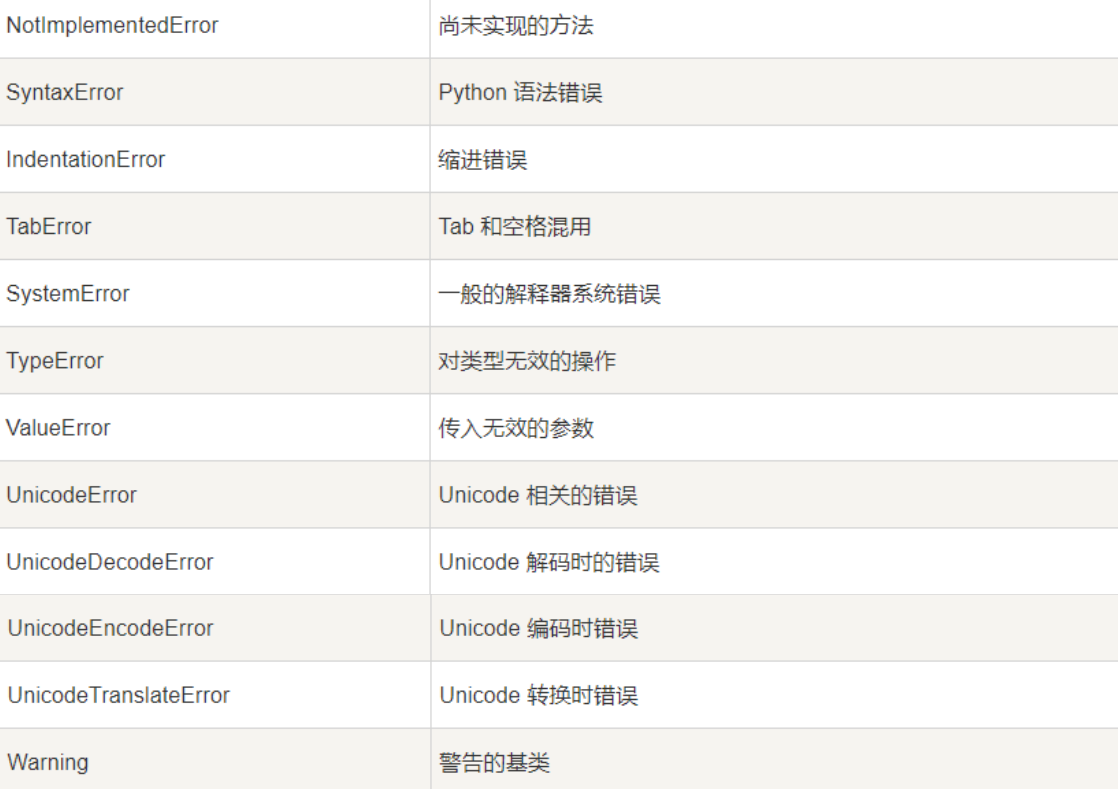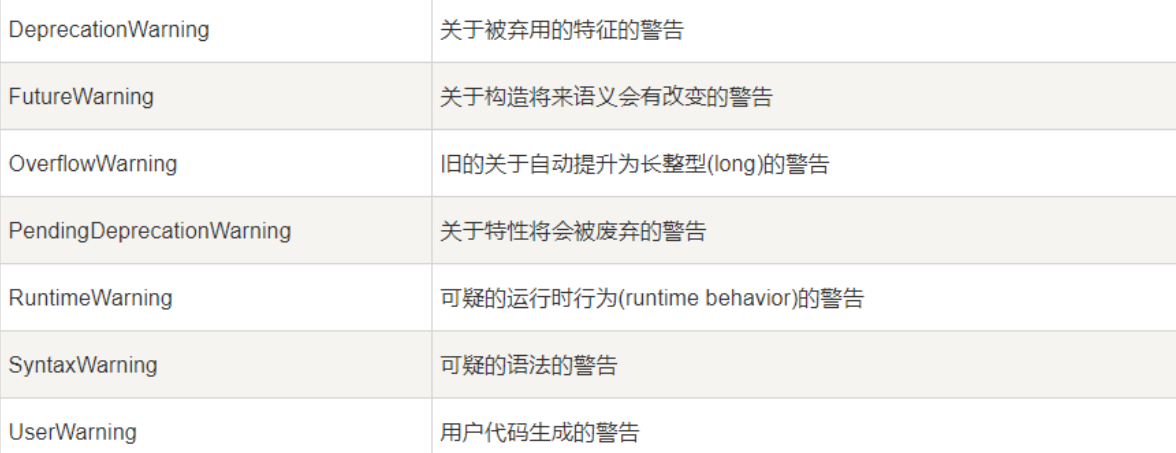5 0

pdf(new) 更多>